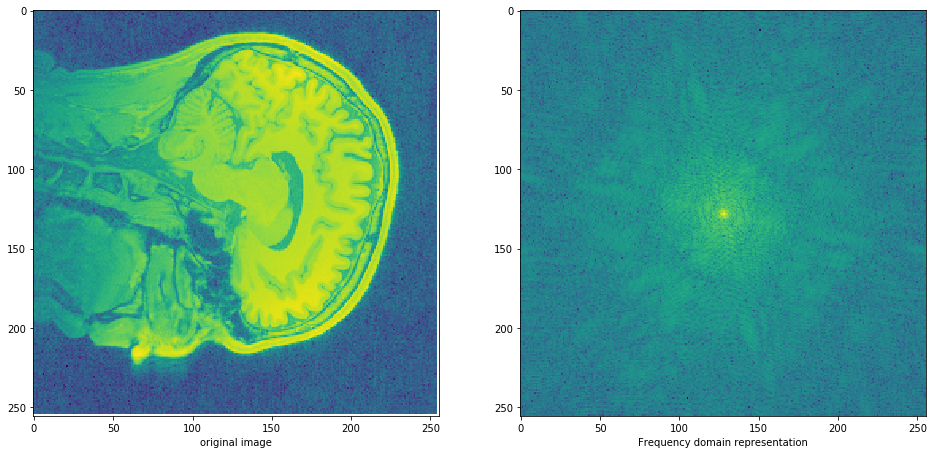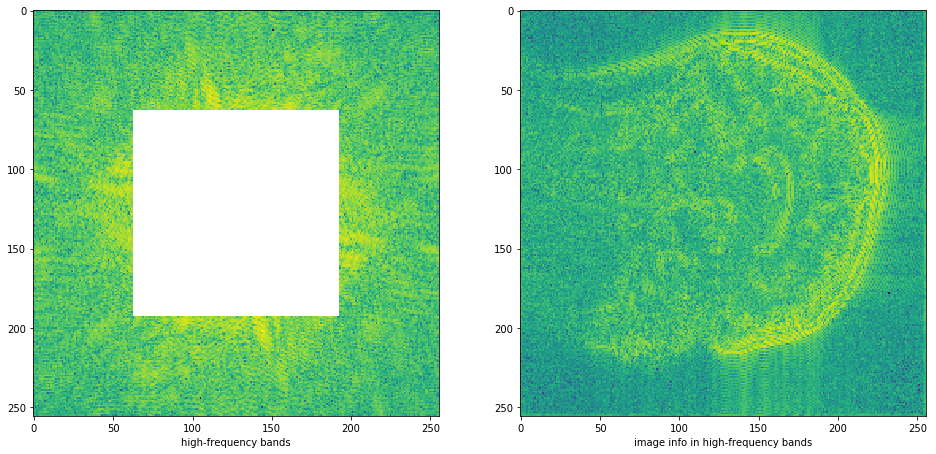### This is a demonstration of aliasing on brain images using slicing.¶

#### Updated 20180519¶

When images are downsampled, any frequency components present in the original image about the downsampled Nyquist frequence gets aliased into the lower frequences. Therefore, images are often processed with an anti-aliasing filter that removes frequency components above the new Nyquist frequency. This anti-aliasing filter can be constructed using many existing filter design methods.

When an image in numpy array is subsampled using slicing:

new_img = img[::2, ::2]

then this can suffer from aliasing issues. When an image needs slicing is dependent on how the image was sampled. For example, in an EPI-volume, o

In :
%matplotlib inline
import matplotlib.pyplot as plt

import numpy as np
import nibabel as nb


# Part 1: Brain slice example¶

## (scroll to Part 2)¶

In :
img = nb.load('T1.nii.gz')

In :
data = img.get_data()
data.shape

Out:
(176, 256, 256)
In :
slice = data[78, :, :]
slice_f = np.fft.fft2(slice)


Let's plot the frequency domain representation of this image. We are plotting on a log-scale to highlight image features.

In :
fig, ax = plt.subplots(1, 2, figsize=(16, 8))
ax.imshow(np.log10(slice), interpolation='nearest')
ax.set_xlabel('original image')
ax.imshow(np.log10(np.abs(np.fft.fftshift(slice_f))), interpolation='nearest')
ax.set_xlabel('Frequency domain representation');

/software/miniconda3/envs/aliasing/lib/python3.6/site-packages/ipykernel_launcher.py:2: RuntimeWarning: divide by zero encountered in log10Now we can remove the central chunk (the low frequency components if we were downsampling by 2) and look at what information is contained in the remaining higher frequency bands.

In :
slice_f_inner = np.fft.fftshift(slice_f)
slice_f_inner[63:193, 63:193] = 0
slice_highfreq = np.abs(np.fft.ifft2(np.fft.fftshift(slice_f_inner)))

fig, ax = plt.subplots(1, 2, figsize=(16, 8))
ax.imshow(np.log10(np.abs(slice_f_inner)), interpolation='nearest')
ax.set_xlabel('high-frequency bands')
ax.imshow(np.log10(slice_highfreq), interpolation='nearest')
ax.set_xlabel('image info in high-frequency bands');

/software/miniconda3/envs/aliasing/lib/python3.6/site-packages/ipykernel_launcher.py:6: RuntimeWarning: divide by zero encountered in log10Use a very coarse anti-aliasing filter by removing the outer chunk of the frequency representation.

In :
slice_sub2 = slice[::2, ::2]
slice_f_outer = np.fft.fftshift(slice_f)
slice_f_outer[0:70, :] = 0
slice_f_outer[186:, :] = 0
slice_f_outer[:, 0:70] = 0
slice_f_outer[:, 186:] = 0
slice_antialiased = np.abs(np.fft.ifft2(np.fft.fftshift(slice_f_outer)))
slice_aa_sub2 = slice_antialiased[::2, ::2]

In :
fig, ax = plt.subplots(1, 2, figsize=(16, 8))
ax.imshow(np.log10(np.abs(slice_f_outer)), interpolation='nearest')
ax.set_xlabel('low-frequency bands')
ax.imshow(np.log10(slice_antialiased), interpolation='nearest')
ax.set_xlabel('image info in low-frequency bands');

/software/miniconda3/envs/aliasing/lib/python3.6/site-packages/ipykernel_launcher.py:2: RuntimeWarning: divide by zero encountered in log10